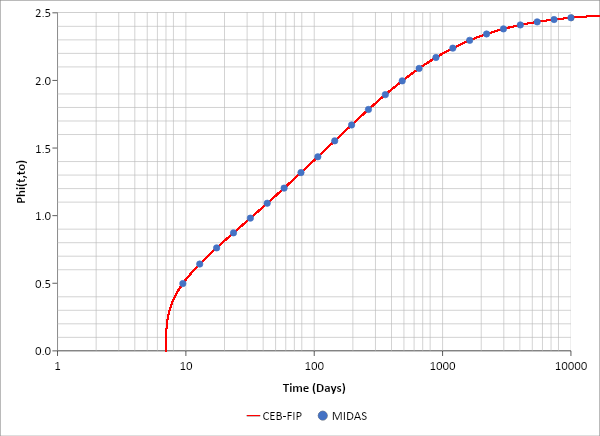GET IT NOW xOverview

The effects of the curvature sometimes can not be ignored in the design process. The following example uses a three-span continuous curved composite steel bridge. You will see a comparison result of the straight beam and the curved beam in this article. It will show you how the curvature may influence the design.Figure 1 Framing Plan

The discussion will be provided for the exterior girder G4. The moment of inertia is assumed to be constant along the bridge, and the ratio of EI/GJ is assumed constant as 300.

The span length ratio is 210/160=1.3125; the side span is 160/700=0.2286, rad=13.10°; and the center span is 210/700=0.3000, rad=17.19°.Figure 2 Bending Moment due to Full Unit Load

Figure 2 shows the complete unit load moment diagram for curved and straight beams with the same span length. At the 3/8 point (side span), the curved girder moment is around 90% of straight girder moments. At the pier location, this ratio isapproximately 124%, at the center span 72%. Even with this medium-span bridge, the moment difference is around 30%.Figure 3 Bending Moment due to Full Unit Load, with MIDAS Results (8 divisions/span)

Figure 3 shows MIDAS results with linear beam elements but curved geometry with eight divisions in each span. The moment distribution is close to exact curved beam results, and the difference is around 2%.Figure 4 Shear due to Full Unit Load

Figure 4 shows the shear force comparison, and the maximum difference is around 6%.Figure 5 Moment Influence Line at 3/8 (End Span)

Figure 5 shows the moment influence line at 3/8 point (side span). The shape itself is similar but the maximum difference is around 33% at the 11/8 point.Figure 6 Moment Influence Line at 8/8 (End Span)

Figure 6 shows the moment influence line at the pier location. The shape itself is similar but the maximum difference is around 32% at 11/8 point.Figure 7 Moment Influence Line at 12/8 (Center Span)

Figure 7 shows the moment influence line at the center-span center location. The shape itself is similar but the maximum difference is around 15% at 12/8 point.

Conclusion

Even in the medium-span curved bridges, the effect of the curvature is more than 30% and can not be ignored in the design. Not many programs can handle this problem, and the users have to understand the limits of each program and apply their engineering judgment on how to proceed.Keep Updated With Technical Contents

Keeping You Updated

You might also like...#### Expert Tips: Creep Analysis 1#### Webinar: 2D Excavation Supported by Tiebacks & Rakers#### Workshop: Application of Boundary Conditions